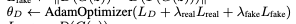# Why can't I accumulate gradients?

Following is snippet from DCGAN pytorch tutorial

``````        netD.zero_grad()
real_cpu = data.to(device)
b_size = real_cpu.size(0)
label = torch.full((b_size,), real_label, dtype=torch.float, device=device)
output = netD(real_cpu).view(-1)
errD_real = criterion(output, label)
errD_real.backward() # <------------------------ BACKWARD CALLED ONCE
D_x = output.mean().item()

noise = torch.randn(b_size, nz, 1, 1, device=device)
fake = netG(noise)
label.fill_(fake_label)
output = netD(fake.detach()).view(-1)
errD_fake = criterion(output, label)
errD_fake.backward() # <------------------------ BACKWARD CALLED AGAIN
D_G_z1 = output.mean().item()
errD = errD_real + errD_fake
optimizerD.step()
``````

Here backward is called twice with no issue. Gradients are “accumulated” (according to the tutorial) and are used to update the weights with `optimizerD.step`

However when I did the following

``````        D_x = netD(x)
D_T_x = netD(T_x)
errD_real = criterion(D_x, label)
errD_real.backward() # <------------------------ BACKWARD CALLED ONCE
L_real = l2loss(D_x, D_T_x)
L_real.backward() # <------------------------ BACKWARD CALLED AGAIN
``````

I get this error

``````RuntimeError: Trying to backward through the graph a second time (or directly access saved variables after they have already been freed). Saved intermediate values of the graph are freed when you call .backward() or autograd.grad(). Specify retain_graph=True if you need to backward through the graph a second time or if you need to access saved variables after calling backward.
``````

And I don’t see why the former is okay but the latter isn’t when they should be concerning the same graph for the netD parameters.

Best,
Gordon

The key bit is that you have the `Dx = netD(x)` evaluation as a grad-requiring bit that would be backwarded through. Note how the the example you cite uses `output = netD(fake.detach()).view(-1)` before the second backward. It detaches fake, so the backward doesn’t go through the `netG` computation and then computes the `netD` evaluation which has not been used in the first backward (which has its own `netD` evaluation).

If you don’t have much computation between the two backwards (if l2loss is what it sounds like) and want to accumulate grads for both losses in the `netD` parameters, maybe doing the backward through the sum of the two losses is a good option. (It should be more efficient than using `retain_graph=True` in the first backward, although that would work, too.)

Best regards

Thomas

1 Like

That makes perfect sense especially since the pseducode did in fact used an addition of losses.Much thanks,
Gordon

I’m afraid my understanding isn’t complete yet.

``````        D_x = netD(x)

# bCR: Forward pass augmented real batch through D
D_T_x = netD(T_x)

errD_real = criterion(D_x, label)

# bCR: Calculate L_real: |D(x) − D(T(x))|^2
L_real = l2loss(D_x, D_T_x)

(errD_real + L_real).backward() # <--- backward called once for first netD evaluation

# Format for print
D_x = D_x.mean().item()

# train with fake
z = torch.randn(batch_size, nz, 1, 1, device=device)
G_z = netG(z)

# bCR: Augment generated images
T_G_z = transform(G_z.detach())

label.fill_(fake_label)
D_G_z = netD(G_z.detach())

# bCR: Forward pass augmented fake batch through D
D_T_G_z = netD(T_G_z)

errD_fake = criterion(D_G_z, label)

# bCR: Calculate L_fake: |D(G(z)) − D(T(G(z)))|^2
L_fake = l2loss(D_G_z, D_T_x)

(errD_fake + L_fake).backward() <--- backward called once for second evaluation of netD
``````

The first backward() works but the second one gives me the same error as above. Perhaps my understanding of ‘evaluation of netD’ is wrong. Do you mean evaluation of netD as in netD(x) being one evaluation and netD(y) being another?

Try recomputing `D_T_x` just before computing `L_fake` and see if this fixes this error.

Dang it does! Thanks! You also made me realise that I was using the wrong target anyways. Like its literally in the comment above to use `D_T_G_z`But I gotta ask…are the weights also backprogagated for the target in a loss function? Because the issue seems to be that the gradients were backprogated twice for the same evaluation for D_T_x. If you could help me clear this up, I would deeply appreciate it.

Glad to be of help. This tutorial may help with some of your questions.

1 Like